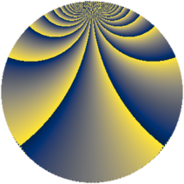# Properties

 Label 2736.3.zLevel $2736$ Weight $3$ Character orbit 2736.z Rep. character $\chi_{2736}(683,\cdot)$ Character field $\Q(\zeta_{4})$ Dimension $640$ Sturm bound $1440$

# Related objects

## Defining parameters

 Level: $$N$$ $$=$$ $$2736 = 2^{4} \cdot 3^{2} \cdot 19$$ Weight: $$k$$ $$=$$ $$3$$ Character orbit: $$[\chi]$$ $$=$$ 2736.z (of order $$4$$ and degree $$2$$) Character conductor: $$\operatorname{cond}(\chi)$$ $$=$$ $$912$$ Character field: $$\Q(i)$$ Sturm bound: $$1440$$

## Dimensions

The following table gives the dimensions of various subspaces of $$M_{3}(2736, [\chi])$$.

Total New Old
Modular forms 1936 640 1296
Cusp forms 1904 640 1264
Eisenstein series 32 0 32

## Trace form

 $$640q + O(q^{10})$$ $$640q + 112q^{16} + 64q^{19} + 4480q^{49} - 128q^{61} - 96q^{64} + 336q^{76} - 400q^{82} - 640q^{85} + O(q^{100})$$

## Decomposition of $$S_{3}^{\mathrm{new}}(2736, [\chi])$$ into newform subspaces

The newforms in this space have not yet been added to the LMFDB.

## Decomposition of $$S_{3}^{\mathrm{old}}(2736, [\chi])$$ into lower level spaces

$$S_{3}^{\mathrm{old}}(2736, [\chi]) \cong$$ $$S_{3}^{\mathrm{new}}(912, [\chi])$$$$^{\oplus 2}$$